### Mathematica Lab Tutorials: Lab 1

### Lab questions

This lab covers Variables, Matrices, Built-in Functions, and Plotting:

1. Consider the relationship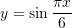Find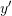and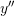. Evaluate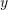,, andat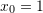. Then, plot the relationships,, andfor the domain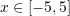. Label your plot and plot legend appropriately.

2. Consider the sequence whose terms are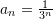. Evaluate the sum of the first twenty terms. Then, evaluate the infinite sum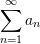Use the ListPlot command to plot the first ten terms of the ordered pairs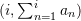. Label the plot appropriately and use the option “Joined->True”.

3. Consider the matrix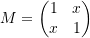Plot the relationship between the determinant of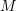andfor. Label the plot appropriately. From the plot, can you identify the values offor which the determinant is equal to zero?